# Checking Whether Cells in a Range are Blank, and Counting the Blank Cells

### Count Blank Cells Using COUNTBLANK

Assume you have this data.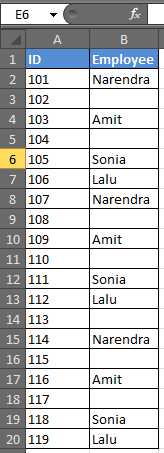Let’s just step into the example.

# How To Check If A Cell Is Blank Using IF

Generic Formula

In the above data, there are some names that are missing. In Column C you want TRUE if it doesn’t have a name and FALSE if it does. It is easy to do this. In C2 write this formula and copy it in the cells below.

 =IF(B2="",TRUE,FALSE)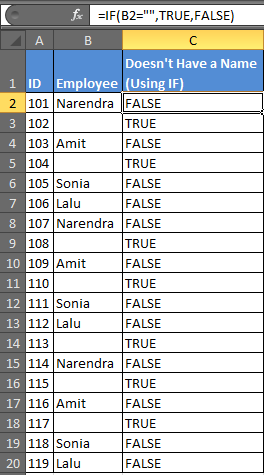The formula simply checks to see if the cell is blank or not. It uses “”  to indicate blank. Prints TRUE  if it is blank, and FALSE if not.

# How To Check If A Cell Is Blank Using ISBLANK

Generic Formula

Now the same task can be done easily by using the ISBLANKfunction in excel 2016.

ISBLANK takes only one argument and that is the cell you want to check.

In D2 write this formula and copy it below cells.

 =ISBLANK(B2)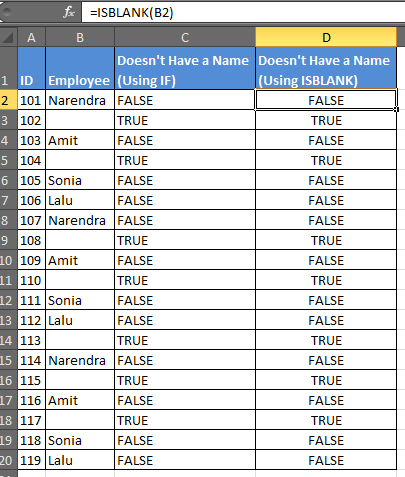You got the same answers by giving just one argument in ISBLANK.

Mostly you would want to do something after knowing if the cell is blank or not. This is where IF is necessary.

For example, if you want to print the name Cells of B column has one, and “mystry_man” if it is a blank cell. You can use IF and ISBLANK in excel together.

In Range E2, write this formula:

 =IF(ISBLANK(B2),"Mystry_Man",B2)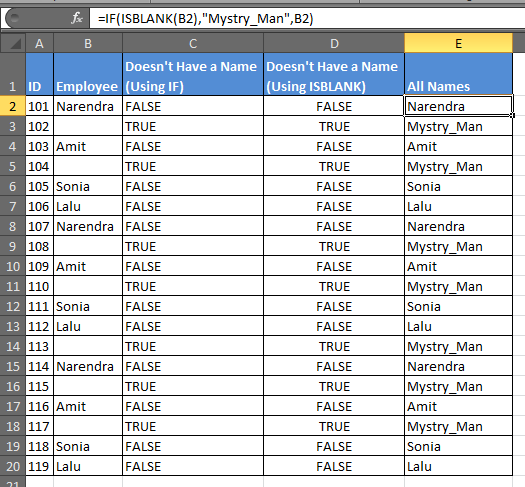# Count Blank Cells Using COUNTIF

Generic Formula

 =COUNTIF(range,””)

Now if you want to know how many blank cells there are, you can use this formula.

In any version of Excel 2016, 2013, 2010, write this formula in any cell.

 =COUNTIF(B2:B20,"")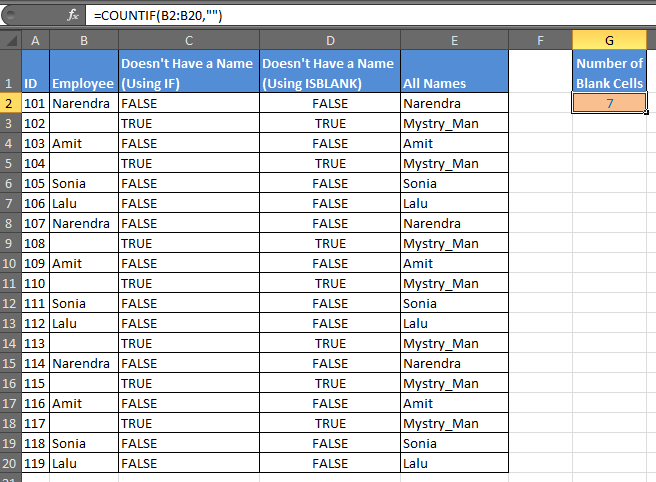We have our answer as 7 for this example.

Now if you want to know how many names there are, you need to count if not blank. Use the COUNTA function to count non-blank cells in a range.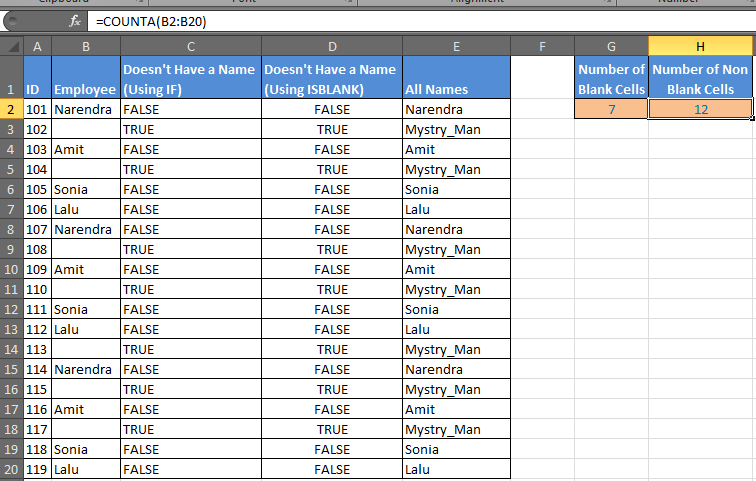# Count Blank Cells Using COUNTBLANK

Generic Formula

 =COUNTBLANK(range)

COUNTA counts if cell contains any text and the COUNTBLANK function counts all blank cells in a range in Excel. It only requires one argument to count empty cells in a range.

In cell G2, enter this formula:

 =COUNTBLANK(B2:B20)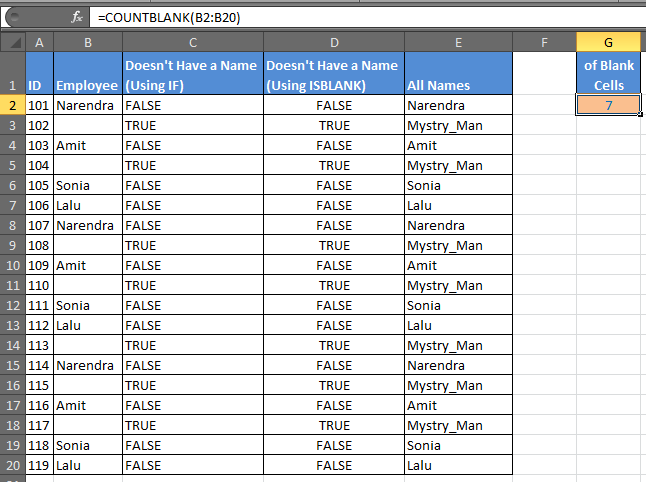COUNTBLANK will find blank cells in excel of the given range.

We learned how to work with blank cells in excel, how to find blank cells, how to count blank cells in a range, how to check if a cell is empty or not, how to manipulate blank cells etc.

Related Articles:

How to Calculate Only If Cell is Not Blank in Excel

Adjusting a Formula to Return a Blank

Checking Whether Cells in a Range are Blank, and Counting the Blank Cells

SUMIF with non-blank cells

Only Return Results from Non-Blank Cells

Popular Articles:

50 Excel Shortcuts to Increase Your Productivity

How to use the VLOOKUP Function in Excel

How to use the COUNTIF function in Excel

How to use the SUMIF Function in Excel

Terms and Conditions of use

The applications/code on this site are distributed as is and without warranties or liability. In no event shall the owner of the copyrights, or the authors of the applications/code be liable for any loss of profit, any problems or any damage resulting from the use or evaluation of the applications/code.